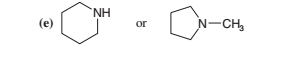# Problem: Which compound in each of the following pairs would have the higher boiling point? Explain your answers.

###### Problem Details

Which compound in each of the following pairs would have the higher boiling point? Explain your answers.What scientific concept do you need to know in order to solve this problem?

Our tutors have indicated that to solve this problem you will need to apply the Physical Properties of Amines concept. If you need more Physical Properties of Amines practice, you can also practice Physical Properties of Amines practice problems.

What is the difficulty of this problem?

Our tutors rated the difficulty ofWhich compound in each of the following pairs would have the...as low difficulty.

How long does this problem take to solve?

Our expert Organic tutor, Chris took 1 minute and 50 seconds to solve this problem. You can follow their steps in the video explanation above.

What textbook is this problem found in?

Our data indicates that this problem or a close variation was asked in Organic Chemistry - Solomons 10th Edition. You can also practice Organic Chemistry - Solomons 10th Edition practice problems.